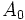Non-normal subgroups of dihedral group:D8

This article is about a particular subgroup in a group, up to equivalence of subgroups (i.e., an isomorphism of groups that induces the corresponding isomorphism of subgroups). The subgroup is (up to isomorphism) cyclic group:Z2 and the group is (up to isomorphism) dihedral group:D8 (see subgroup structure of dihedral group:D8).
VIEW: Group-subgroup pairs with the same subgroup part | Group-subgroup pairs with the same group part | All pages on particular subgroups in groups

Definition

Suppose$G$ is the dihedral group of order eight (degree four) given by the presentation below, where$e$ denotes the identity element of$G$:$G := \langle a,x \mid a^4 = x^2 = e, xax = a^{-1} \rangle$.

Then, we are interested in the following four subgroups:$A_0 := \langle x \rangle = \{ x, e \}, A_1 := \langle ax \rangle = \{ ax, e \}, A_2 := \langle a^2x \rangle = \{ a^2x, e \}, A_3 = \langle a^3x \rangle = \{ a^3x, e \}$.$A_0$ and$A_2$ are conjugate subgroups (via$a$, for instance).$A_1$ and$A_3$ are conjugate subgroups (via$a$, for instance).$A_0$ and$A_1$ are not conjugate but are related by an outer automorphism that fixes$a$ and sends$x$ to$ax$. Thus, all four subgroups are automorphic subgroups. These are the only non-normal subgroups of$G$ and they are all 2-subnormal subgroups.

Arithmetic functions

Function Value Explanation
order of whole group 8
order of subgroup 2
index 2
size of conjugacy class 2
number of conjugacy classes in automorphism class 2
size of automorphism class 2
subnormal depth 2
hypernormalized depth 2

Effect of subgroup operators

Specific values (in the second column) are for$A_0 = \langle x \rangle$.

Function Value as subgroup (descriptive) Value as subgroup (link) Value as group
normalizer$\langle a^2, x \rangle$ Klein four-subgroups of dihedral group:D8 Klein four-group
centralizer$\langle a^2,x \rangle$ Klein four-subgroups of dihedral group:D8 Klein four-group
normal core$\{ e \}$ -- trivial group
normal closure$\langle a^2,x \rangle$ Klein four-subgroups of dihedral group:D8 Klein four-group
characteristic core$\{ e \}$ -- trivial group
characteristic closure$G$, i.e.,$\langle a,x \rangle$ -- dihedral group:D8

Related subgroups

Intermediate subgroups

We use$A_0 = \langle x\rangle$ here.

Value of intermediate subgroup (descriptive) Isomorphism class of intermediate subgroup Small subgroup in intermediate subgroup Intermediate subgroup in big group$\langle a^2, x \rangle$ Klein four-group Z2 in V4 Klein four-subgroups of dihedral group:D8

Subgroup properties

Invariance under automorphisms and endomorphisms

Suppose$c_a$ and$c_x$ denote conjugation by$a$ and$x$ respectively. Let$\sigma$ denote the automorphism that sends$a$ to$a^3$ and$x$ to$ax$. Then,$\langle c_a, c_x\rangle$ is the inner automorphism group and$\langle c_a, c_x, \sigma \rangle$ is the automorphism group.

The automorphism$c_x$ fixes$A_0$ and$A_2$ while interchanging$A_1$ and$A_3$. The automorphism$c_a$ interchanges$A_0$ and$A_2$ while also interchanging$A_1$ and$A_3$. The automorphism$c_{ax} = c_a \circ c_x$ fixes$A_1$ and$A_3$ while interchanging$A_0$ and$A_2$. The automorphism$\sigma$ interchanges$A_0$ and$A_1$ and also interchanges$A_2$ and$A_3$.

Property Meaning Satisfied? Explanation Comment
normal subgroup invariant under inner automorphisms No See above description of conjugation automorphisms that permute the subgroups
coprime automorphism-invariant subgroup invariant under automorphisms of coprime order to group Yes there are no nontrivial automorphisms of coprime order
cofactorial automorphism-invariant subgroup invariant under all automorphisms whose order has prime factors only among those of the group No follows from not being normal
2-subnormal subgroup normal subgroup of normal subgroup Yes normal inside Klein four-subgroups of dihedral group:D8 (of the form$\langle a^2,x \rangle$ and$\langle a^2,ax\rangle$) that are normal in the whole group.
subnormal subgroup Yes follows from being 2-subnormal, also from being subgroup of nilpotent group.